# Converting Percents To Fractions

in STEMGeekslast year

Hi there. In this post, I cover the topic of converting percents into fractions. It is assumed that the reader/student is comfortable with multiplication, division and multiplying fractions.

Math text rendered with Quicklatex.comPixabay Image Source

## One Percent As One Out Of 100

The concept of a percent means to take a part of something. One percent is one out of 100. As a fraction, 1 percent is expressed as 1/100.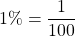Five percent would be 5 out of 100 which can be reduced to 1 out of 20 from divided both 5 and 100 by 5.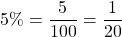Ten percent would be 10 out of 100 which can be reduced to 1 out of 10.

Other Common Percentages With Their Equivalent Fractions

PercentageFraction
20%1/5
25%1/4
50%1/2
75%3/4
100%1/1 or just 1 as in 1 whole

## Percents Above 100%

There are cases where percentages are above 100%. It looks scary when it comes to dealing with such percentages but it is not that bad.

In general we have: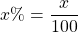Something like 200% would be 200 out of 100. This 200 out of 100 can be seen as 200 divided by 100 which is just 2.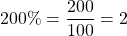Having 750% would be 7 and a half.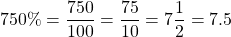Pixabay Image Source

## Percentages With Decimals

There are cases where percentages do contain decimals. Converting these percentages into fractions does take a little bit more work. Keep in mind that fractions do not typically contain decimals and the fractions should have whole numbers in the top (numerator) and the bottom (denominator).

Example One

Expressing 1.1% as a fraction.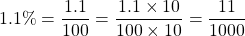Note that I multiply both 1.1 and 100 by 10 to make 1.1 into 11 and the 100 into 1000.

Example Two

Expressing 120.7% as a fraction.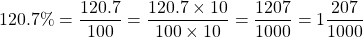Pixabay Image Source

## Keyword Of Means Multiply

The previous sections dealt with just the percents itself. When the keyword of is included you are taking a percentage of something. Another way to think about this is taking a portion/part of something. This is where multiplying fractions comes in.

Example One

What is 10% of 1200?

Before the setup, note that 10% is 10 out of 100 or 1 out of 10. The keyword of means multiply. We have the following calculations.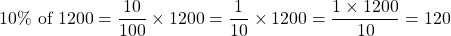Example Two

Determine the amount of 210% of 24.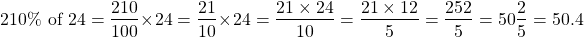Example Three

How much is 2.3% of 88?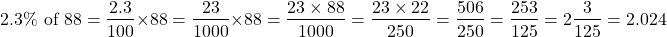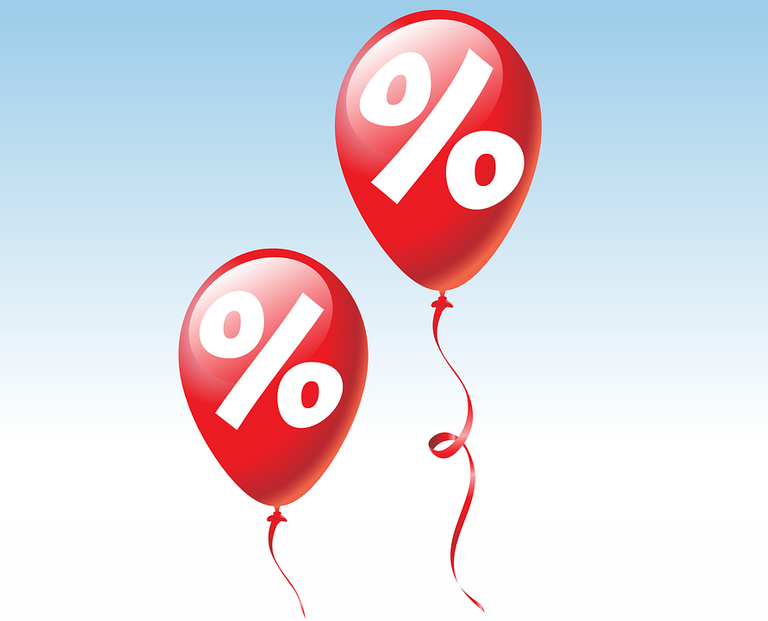Pixabay Image Source